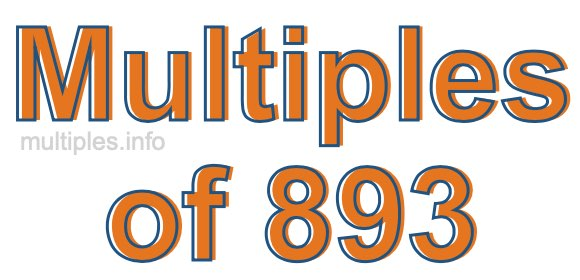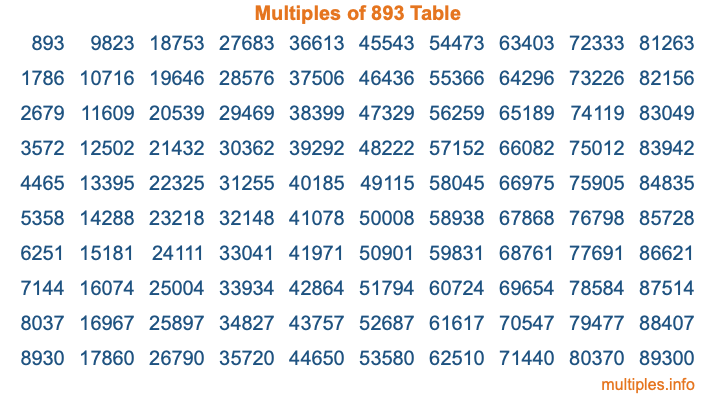Multiples of 893Welcome to the Multiples of 893 page. Here we will first teach you everything you will ever need to know about the multiples of 893, and then give you a study guide summary of everything we taught you to make sure you remember it all. Use this page to look up facts and learn information about the multiples of 893. This page will make you a multiples of eight hundred ninety-three expert!

Definition of Multiples of 893
Multiples of 893 are all the numbers that when divided by 893 equal an integer. Each of the multiples of 893 are called a multiple. A multiple of 893 is created by multiplying 893 by an integer.

Therefore, to create a list of multiples of 893, you start with 1 multiplied by 893, then 2 multiplied by 893, then 3 multiplied by 893, and so on for as long as you want. Thus, the list of the first five multiples of 893 is 893, 1786, 2679, 3572, and 4465. To see a larger list of multiples of 893, see the printable image of Multiples of 893 further down on this page. We also have a category where you can choose any nth multiple of 893.

Multiples of 893 Checker
The Multiples of 893 Checker below checks to see if any number of your choice is a multiple of 893. In other words, it checks to see if there is any number (integer) that when multiplied by 893 will equal your number. To do that, we divide your number by 893. If the the quotient is an integer, then your number is a multiple of 893.

Is  a multiple of 893?

Least Common Multiple of 893 and ...
A Least Common Multiple (LCM) is the lowest multiple that two or more numbers have in common. This is also called the smallest common multiple or lowest common multiple and is useful to know when you are adding our subtracting fractions. Enter one or more numbers below (893 is already entered) to find the LCM.

Check out our LCM Calculator if you need more details about the Least Common Multiple or if you need the LCM for different numbers for adding and subtraction fractions.

nth Multiple of 893
As we stated above, 893 is the first multiple of 893, 1786 is the second multiple of 893, 2679 is the third multiple of 893, and so on. Enter a number below to find the nth multiple of 893.

th multiple of 893

Multiples of 893 vs Factors of 893
893 is a multiple of 893 and a factor of 893, but that is where the similarities end. All postive multiples of 893 are 893 or greater than 893. All positive factors of 893 are 893 or less than 893.

Below is the beginning list of multiples of 893 and the factors of 893 so you can compare:

Multiples of 893: 893, 1786, 2679, 3572, 4465, etc.

Factors of 893: 1, 19, 47, 893

As you can see, the multiples of 893 are all the numbers that you can divide by 893 to get a whole number. The factors of 893, on the other hand, are all the whole numbers that you can multiply by another whole number to get 893.

It's also interesting to note that if a number (x) is a factor of 893, then 893 will also be a multiple of that number (x).

Multiples of 893 vs Divisors of 893
The divisors of 893 are all the integers that 893 can be divided by evenly. Below is a list of the divisors of 893.

Divisors of 893: 1, 19, 47, 893

The interesting thing to note here is that if you take any multiple of 893 and divide it by a divisor of 893, you will see that the quotient is an integer.

Multiples of 893 Table
Below is an image of the first 100 multiples of 893 in a table. The table is in chronological order, column by column. The first column has the first ten multiples of 893, the second column has the next ten multiples of 893, and so on.The Multiples of 893 Table is also referred to as the 893 Times Table or Times Table of 893. You are welcome to print out our table for your studies.

Negative Multiples of 893
Although not often discussed or needed in math, it is worth mentioning that you can make a list of negative multiples of 893 by multiplying 893 by -1, then by -2, then by -3, and so on, to get the following list of negative multiples of 893:

-893, -1786, -2679, -3572, -4465, etc.

Multiples of 893 Summary
Below is a summary of important Multiples of 893 facts that we have discussed on this page. To retain the knowledge on this page, we recommend that you read through the summary and explain to yourself or a study partner why they hold true.

There are an infinite number of multiples of 893.

A multiple of 893 divided by 893 will equal a whole number.

893 divided by a factor of 893 equals a divisor of 893.

The nth multiple of 893 is n times 893.

The largest factor of 893 is equal to the first positive multiple of 893.

893 is a multiple of every factor of 893.

893 is a multiple of 893.

A multiple of 893 divided by a divisor of 893 equals an integer.

893 divided by a divisor of 893 equals a factor of 893.

Any integer times 893 will equal a multiple of 893.

Multiples of a Number
Here you can get the multiples of another number, all with the same attention to detail as we did for multiples of 893 on this page.

Multiples of
Multiples of 894
Did you find our page about multiples of eight hundred ninety-three educational? Do you want more knowledge? Check out the multiples of the next number on our list!